Categories

## 🎨 gifcast Color Profiles

gifcast—discussed in a prior post—now supports color profile selection.

The following animated GIFs—generated with gifcast—show a sample of available profiles.

Here is the asciinema cast file used to generate the animated GIFs: profile_demo.cast

Categories

## ▶️ gifcast

I implemented gifcast, a web page for converting asciinema casts to animated GIFs. Here’s the link:
https://dstein64.github.io/gifcast/

The JavaScript source code is available on GitHub:
https://github.com/dstein64/gifcast

The example below was generated with gifcast.

Here is the asciinema cast file used to generate the animated GIF: gifcast.cast

Categories

## Compressing VGG for Style Transfer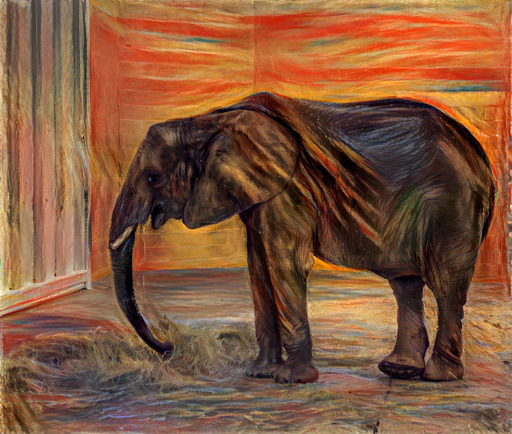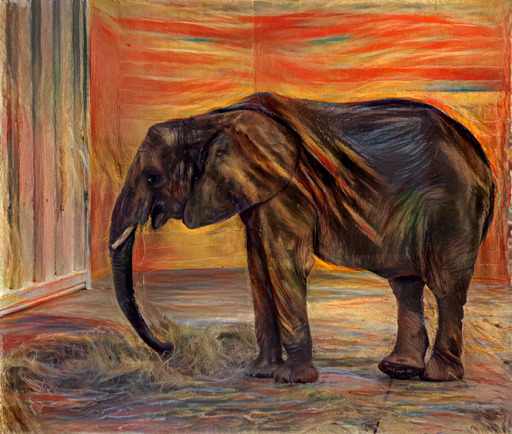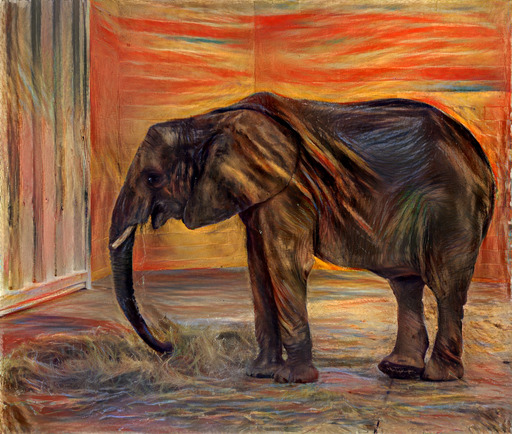32-bit float (no quantization) 8-bit 7-bit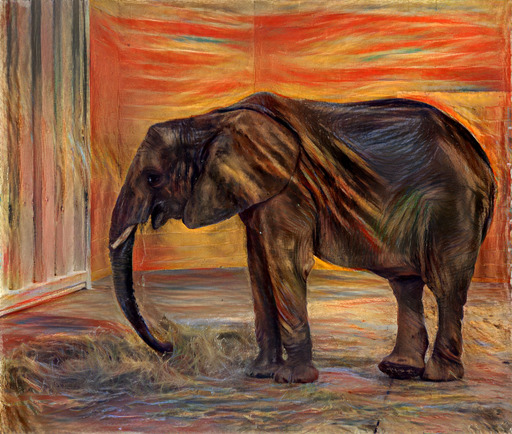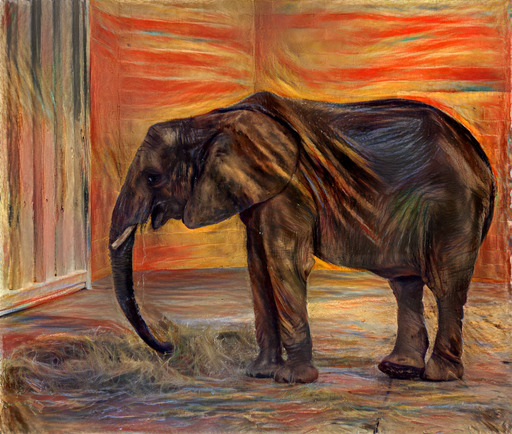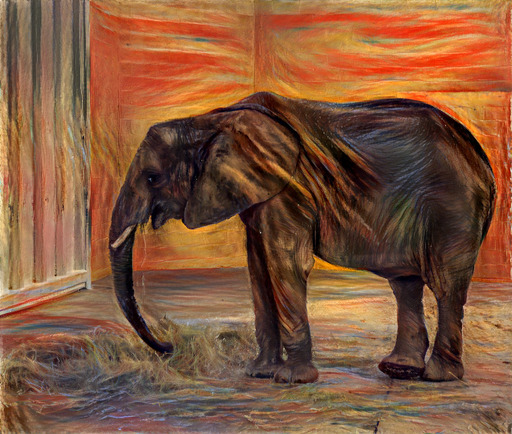6-bit 5-bit 4-bit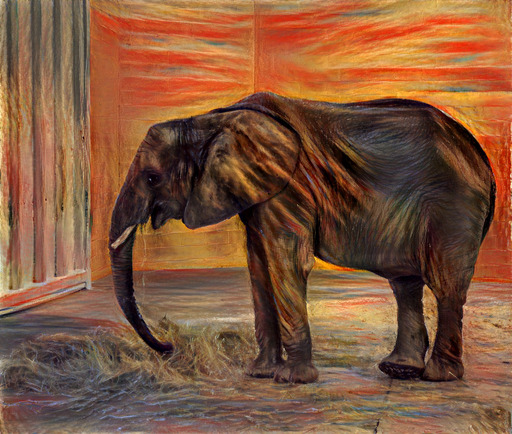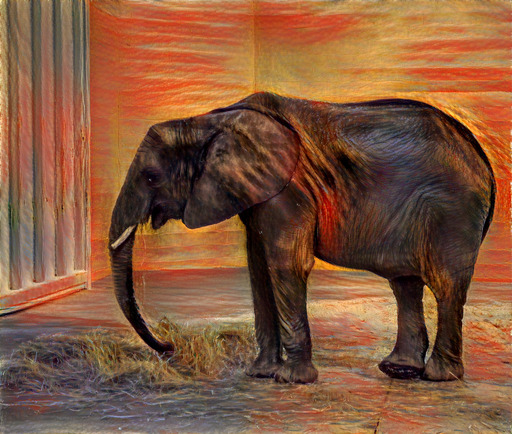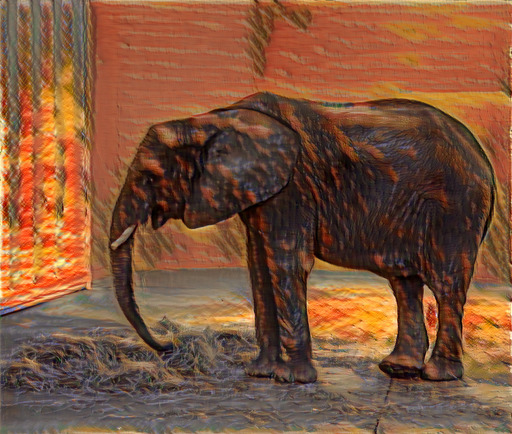3-bit 2-bit 1-bit

I recently implemented pastiche—discussed in a prior post—for applying neural style transfer. I encountered a size limit when uploading the library to PyPI, as a package cannot exceed 60MB. The 32-bit floating point weights for the underlying VGG model  were contained in an 80MB file. My package was subsequently approved for a size limit increase that could accommodate the VGG weights as-is, but I was still interested in compressing the model.

Various techniques have been proposed for compressing neural networks—including distillation  and quantization [3,4]—which have been shown to work well in the context of classification. My problem was in the context of style transfer, so I was not sure how model compression would impact the results.

#### Experiments

I decided to experiment with weight quantization, using a scheme where I could store the quantized weights on disk, and then uncompress the weights to full 32-bit floats at runtime. This quantization scheme would allow me to continue using my existing code after the model is loaded. I am not targeting environments where memory is a constraint, so I was not particularly interested in approaches that would also reduce the model footprint at runtime. I used kmeans1d—discussed in a prior post—for quantizing each layer’s weights.

Categories

## SMAWK in C++

I recently implemented kmeans1d—discussed in a prior post—for efficiently performing globally optimal 1D k-means clustering. The implementation utilizes the SMAWK algorithm (Aggarwal et al., 1987), which calculates `argmin(i)` for each row i of an arbitrary `n × m` totally monotone matrix, in `O(m(1 + lg(n/m)))`.

I’ve factored out my SMAWK C++ code into the example below. In general, SMAWK works with an implicitly defined matrix, utilizing a function that returns a value corresponding to an arbitrary position in the matrix. An explicitly defined matrix is used in the example for the purpose of illustration.

The program prints the column indices corresponding to the minimum element of each row in a totally monotone matrix. The matrix is from monge.pdf—a course document that I found online.

Categories

## kmeans1d: Globally Optimal Efficient 1D k‑means Clustering

I implemented kmeans1d, a Python library for performing k-means clustering on 1D data, based on the algorithm from Xiaolin (1991), as presented by Grønlund et al. (2017, Section 2.2).

Globally optimal k-means clustering is NP-hard for multi-dimensional data. Lloyd’s algorithm is a popular approach for finding a locally optimal solution. For 1-dimensional data, there are polynomial time algorithms.

kmeans1d contains an O(kn + n log n) dynamic programming algorithm for finding the globally optimal k clusters for n 1D data points. The code is written in C++—for faster execution than a pure Python implementation—and wrapped in Python.

The source code is available on GitHub:
https://github.com/dstein64/kmeans1d